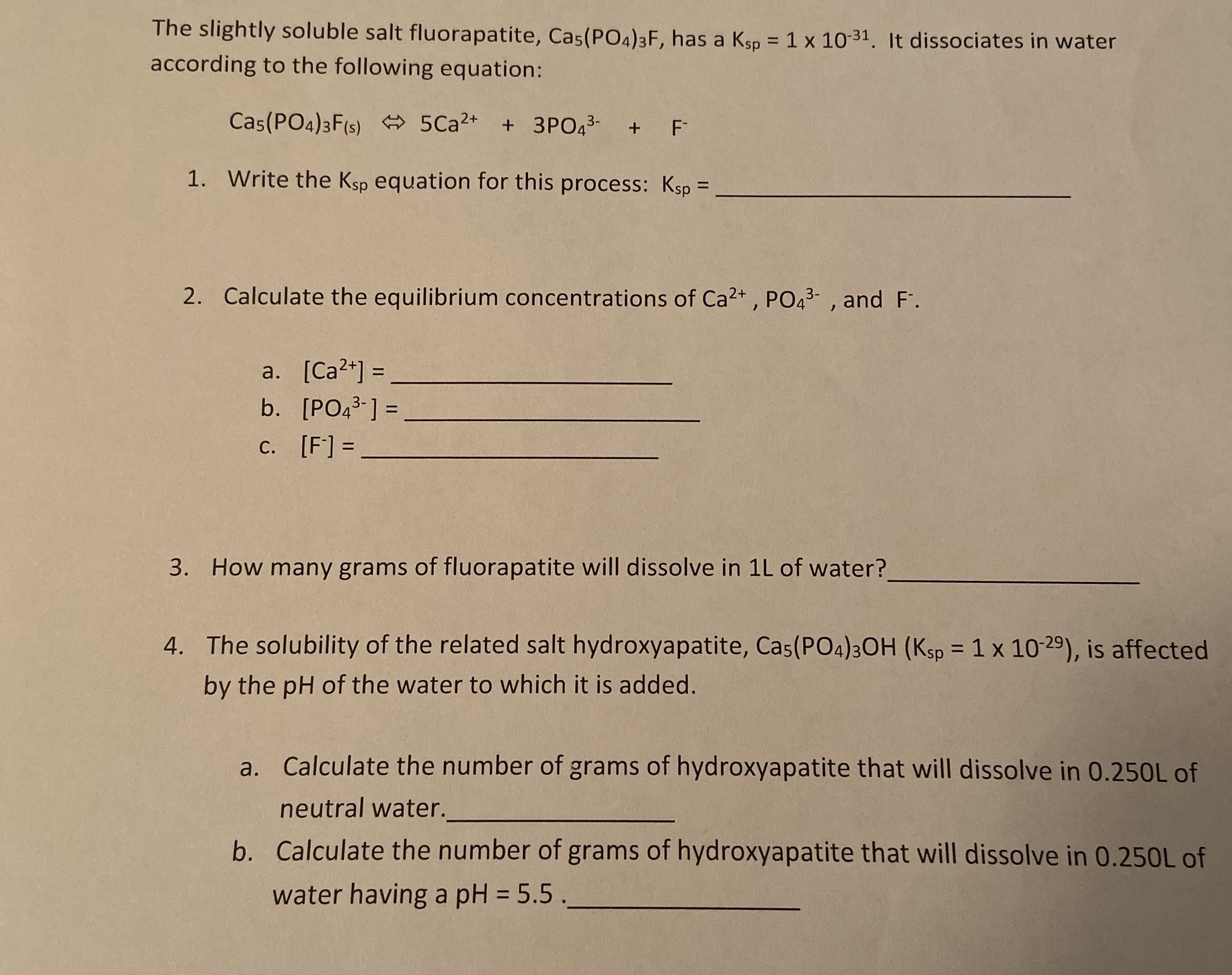# The slightly soluble salt fluorapatite, Cas(PO4)3F, has a Ksp = 1 x 1031. It dissociates in wateraccording to the following equation:Cas(PO4)3F(s) 5Ca2+ +3 PO43-+ FWrite the Ksp equation for this process: Ksp =1.2. Calculate the equilibrium concentrations of Ca2+, PO43- , and F.a. [Ca2] =b. [PO43-]=c. [F] =How many grams of fluorapatite will dissolve in 1L of water?3.4. The solubility of the related salt hydroxyapatite, Cas(PO4)30H (Ksp 1 x 10-29), is affectedby the pH of the water to which it is added.Calculate the number of grams of hydroxyapatite that will dissolve in 0.250L ofa.neutral water.Calculate the number of grams of hydroxyapatite that will dissolve in 0.250L ofb.water having a pH5.5

Question
175 viewshelp_outlineImage TranscriptioncloseThe slightly soluble salt fluorapatite, Cas(PO4)3F, has a Ksp = 1 x 1031. It dissociates in water according to the following equation: Cas(PO4)3F(s) 5Ca2+ +3 PO43- + F Write the Ksp equation for this process: Ksp = 1. 2. Calculate the equilibrium concentrations of Ca2+, PO43- , and F. a. [Ca2] = b. [PO43-]= c. [F] = How many grams of fluorapatite will dissolve in 1L of water? 3. 4. The solubility of the related salt hydroxyapatite, Cas(PO4)30H (Ksp 1 x 10-29), is affected by the pH of the water to which it is added. Calculate the number of grams of hydroxyapatite that will dissolve in 0.250L of a. neutral water. Calculate the number of grams of hydroxyapatite that will dissolve in 0.250L of b. water having a pH 5.5 fullscreen
check_circle

Step 1

The given dissociation re...

### Want to see the full answer?

See Solution

#### Want to see this answer and more?

Solutions are written by subject experts who are available 24/7. Questions are typically answered within 1 hour.*

See Solution
*Response times may vary by subject and question.
Tagged in

### General Chemistry